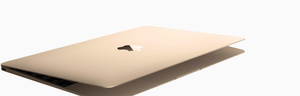## Refurbished Mac

Discover what goes into each refurbished Mac.A\$1,099.00

A\$1,349.00

A\$1,359.00

A\$1,359.00

A\$1,489.00

A\$1,609.00

A\$1,609.00

A\$1,609.00

A\$1,679.00

A\$1,729.00

A\$1,739.00

A\$1,739.00

A\$1,739.00

A\$1,859.00

A\$1,859.00

A\$1,999.00

A\$1,999.00

A\$2,209.00

A\$2,209.00

A\$2,359.00

A\$2,379.00

A\$2,459.00

A\$2,509.00

A\$2,549.00

A\$2,549.00

A\$2,679.00

A\$2,719.00

A\$2,759.00

A\$2,799.00

A\$2,799.00

A\$2,799.00

A\$2,799.00

A\$2,909.00

A\$2,909.00

A\$2,919.00

A\$2,969.00

A\$2,969.00

A\$3,019.00

A\$3,049.00

A\$3,059.00

A\$3,059.00

A\$3,059.00

A\$3,059.00

A\$3,059.00

A\$3,179.00

A\$3,179.00

A\$3,179.00

A\$3,279.00

A\$3,309.00

A\$3,309.00

A\$3,309.00

A\$3,329.00

A\$3,369.00

A\$3,369.00

A\$3,369.00

A\$3,369.00

A\$3,399.00

A\$3,419.00

A\$3,419.00

A\$3,449.00

A\$3,449.00

A\$3,449.00

A\$3,559.00

A\$3,559.00

A\$3,559.00

A\$3,569.00

A\$3,569.00

A\$3,569.00

A\$3,569.00

A\$3,599.00

A\$3,599.00

A\$3,639.00

A\$3,639.00

A\$3,669.00

A\$3,729.00

A\$3,779.00

A\$3,799.00

A\$3,819.00

A\$3,829.00

A\$3,869.00

A\$3,909.00

A\$3,929.00

A\$3,959.00

A\$3,959.00

A\$3,959.00

A\$3,999.00

A\$4,079.00

A\$4,079.00

A\$4,079.00

A\$4,099.00

A\$4,099.00

A\$4,099.00

A\$4,099.00

A\$4,209.00

A\$4,209.00

A\$4,269.00

A\$4,329.00

A\$4,349.00

A\$4,349.00

A\$4,349.00

A\$4,369.00

A\$4,409.00

A\$4,509.00

A\$4,509.00

A\$4,509.00

A\$4,539.00

A\$4,589.00

A\$4,639.00

A\$4,639.00

A\$4,639.00

A\$4,719.00

A\$4,759.00

A\$4,759.00

A\$4,759.00

A\$4,819.00

A\$4,839.00

A\$4,889.00

A\$4,889.00

A\$4,889.00

A\$4,989.00

A\$5,049.00

A\$5,079.00

A\$5,089.00

A\$5,189.00

A\$5,189.00

A\$5,299.00

A\$5,319.00

A\$5,439.00

A\$5,439.00

A\$5,459.00

A\$5,529.00

A\$5,529.00

A\$5,569.00

A\$5,589.00

A\$5,589.00

A\$5,869.00

A\$5,999.00

A\$6,029.00

A\$6,069.00

A\$6,099.00

A\$6,119.00

A\$6,249.00

A\$6,249.00

A\$6,339.00

A\$6,609.00

A\$7,089.00

A\$7,199.00

A\$7,499.00

A\$7,639.00

A\$7,689.00

A\$7,879.00

A\$7,879.00

A\$8,139.00

A\$8,229.00

A\$8,229.00

A\$8,389.00